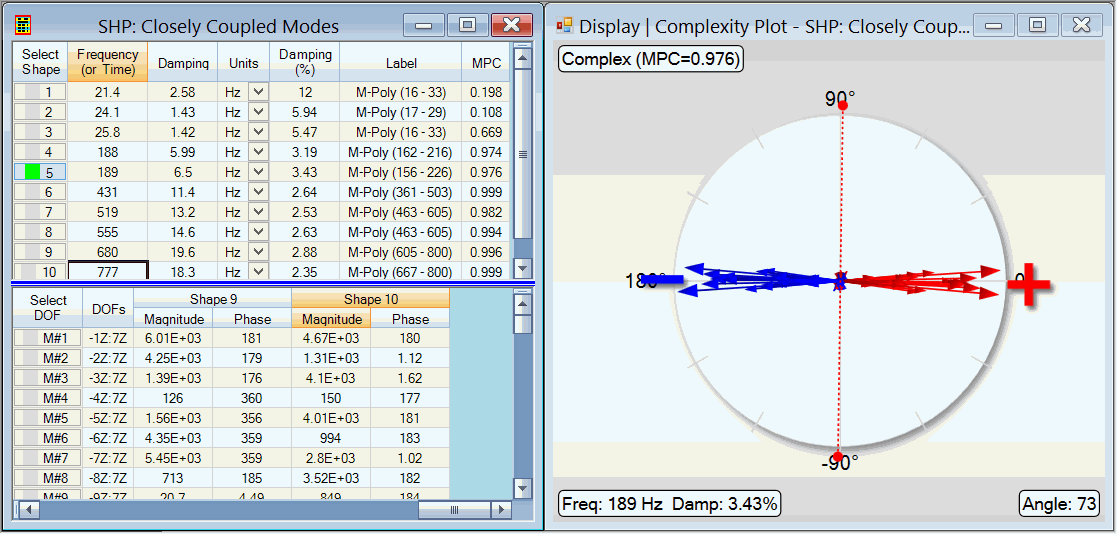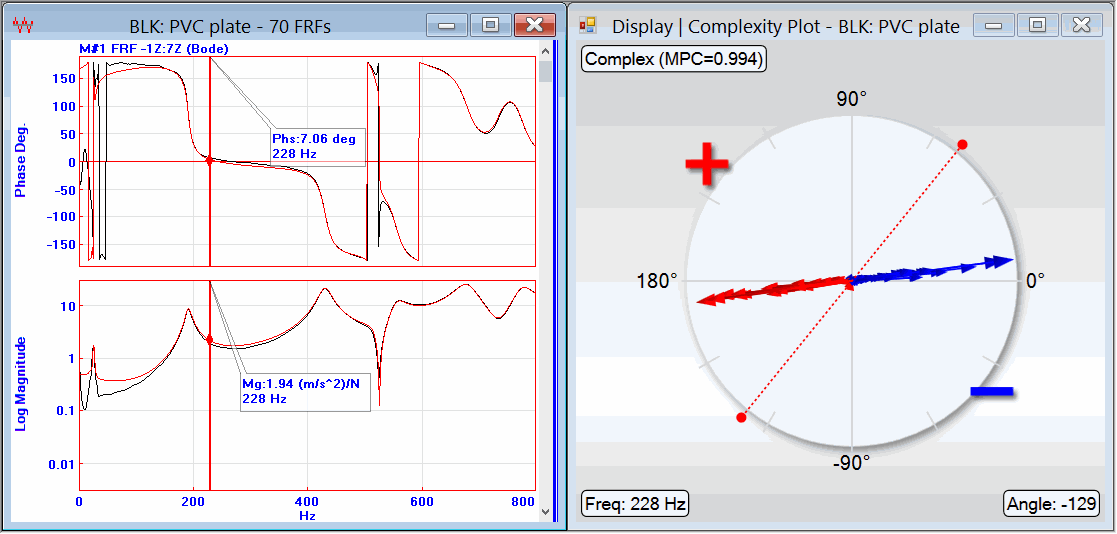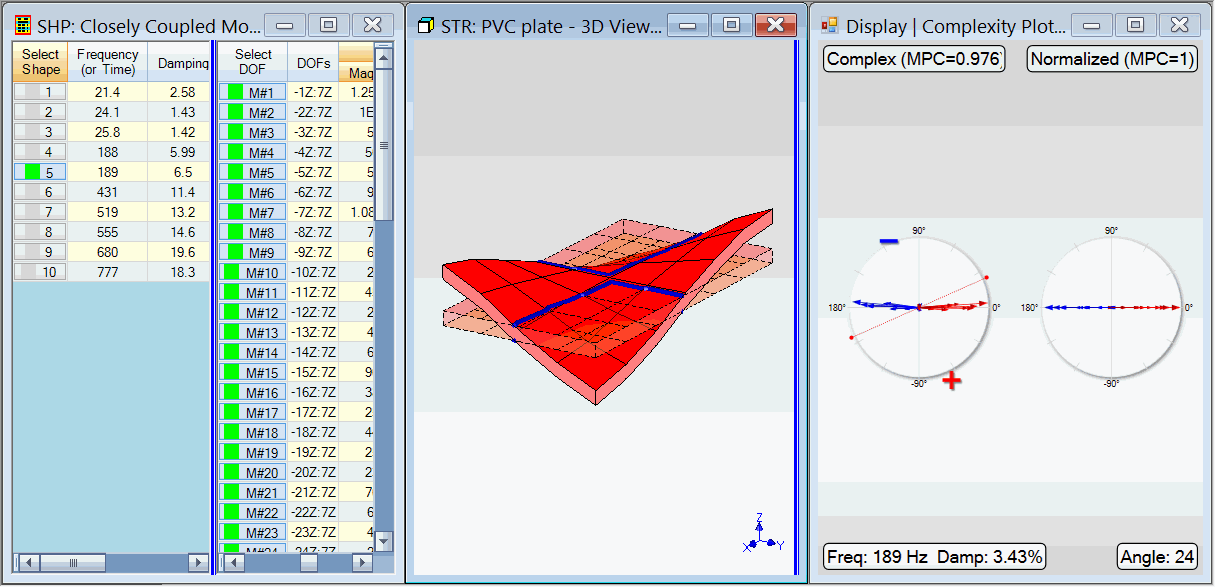# Display | Complexity Plot

Opens the Complexity Plot window.

This command can be executed from either a Data Block or a Shape Table.

## Normal Shape

• Each shape component of a normal shape has a phase of 0 or 180 degrees

• During shape animation, a normal shape exhibits a standing wave motion, and its Node Lines will not move

• The shape components of a normal shape lie on a straight line in a Complexity Plot

An FEA model with no damping yields normal mode shapes.
All phases of a normal mode shape are 0 & 180 degrees.

## Complex Shape

• Each shape component of a complex shape can have an arbitrary phase

• During shape animation, a complex shape can exhibit a traveling wave motion, and its Node Lines can move

• The shape components of a complex shape do not lie on a straight line in a Complexity Plot

Experimental mode shapes can be complex shapes for a number of reasons;

1. Real structures with heavy damping in them can have complex mode shapes

2. Measurement errors can introduce arbitrary phases into the shape component estimates

3. Curve fitting errors can introduce arbitrary phases into the shape component estimates

## Shape Table Complexity Plot

A Shape Table Complexity Plot displays the magnitudes & phases
for all (or selected) shapes
for all (or selected) M#s,Shape Table Complexity Plot.

## Data Block Complexity Plot

A Data Block Complexity Plot displays the magnitudes & phase
all (or selected) M# values
at the current Cursor position.Data Block Complexity Plot.

## Normalized Shapes on the Complexity Plot

When complex shapes are "normalized", they behave like normal shapes.

• When Display | Normalize Shapes is checked, complex shape components are displayed on the left, and normalized shape components are displayed on the right of the Complexity Plot, as shown below

• The normalization line (dashed line) on a Complexity Plot is used to normalize each complex shape.

• When a shape is normalized, the magnitude of each shape component is retained but the phase is changed to either 0 or 180 degrees.

• When a shape is normalized, the red (+) shape components are given 0 degrees phase, and the blue (-) shape components are given 180 degrees phase.

To rotate the normalization line to a different position,

1. Click & drag near the normalization line on the Complexity Plot

2. Or execute Display | Set Normalization Angle, and enter an angle into the dialog box.Complexity Plot with Shape Normalization Turned ON.

## MPC (Modal Phase Colinearity)

If only one shape is displayed in the Complexity Plot, its MPC (Modal Phase Colinearity) value is also displayed.

• MPC values range between 0 &1.

• If MPC = 1, all components the shape lie on a straight line.

• If MPC < 1, some shape components do not lie on a straight line.

• If MPC is "close to 1", this indicates that the structure is lightly damped, or that the mode shape is a normal mode shape.

## Flipping the Phases of the Right-Hand Shape

During Comparison Animation, if two similar shapes appear to be animating 180 degrees out of phase with one another, the phases of right hand shape can be changed by 180 degrees so that the two shapes animate more closely together.

To flip the sign of the right hand shape;

1. Execute Animate | Compare Shapes | Flip Sign in the Structure window to multiply the right hand shape by "-1".

2. Or rotate the normalization line on the Complexity Plot by dragging it to flip the phase of the shape components.